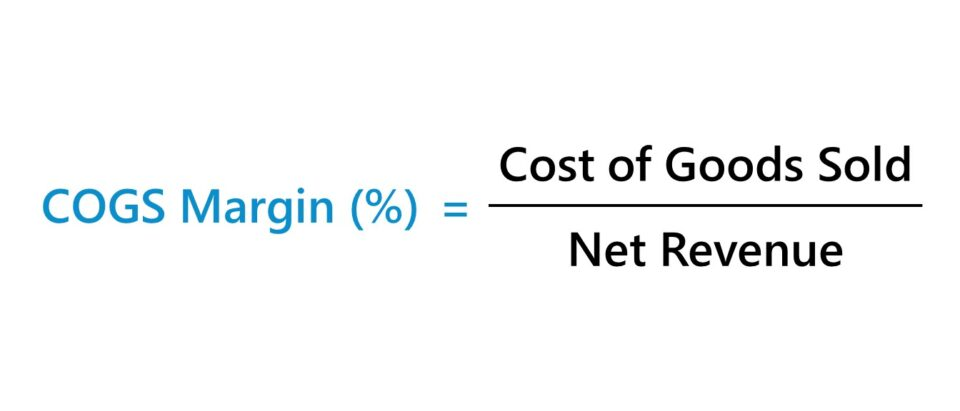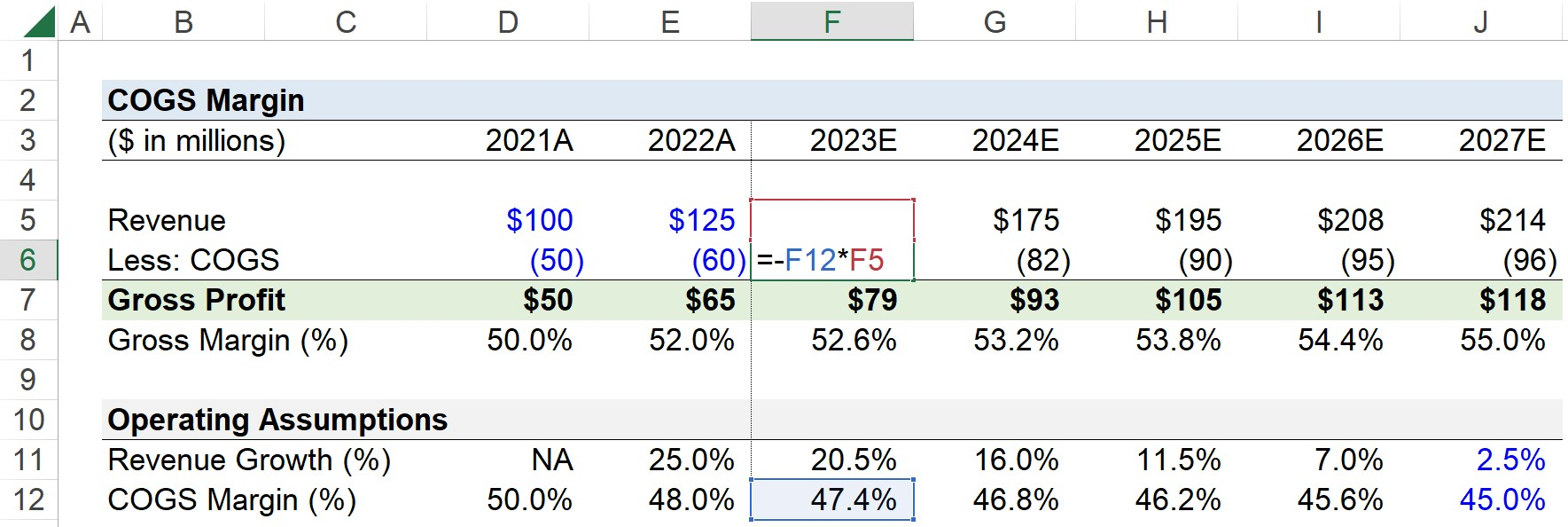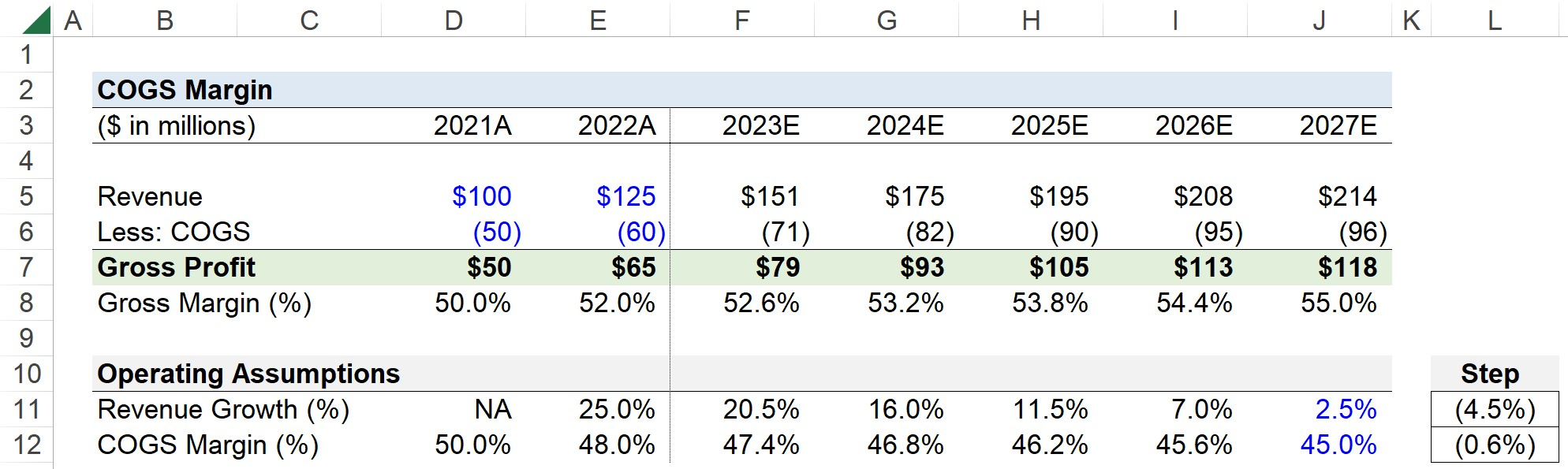# COGS Margin

Guide to Understanding the COGS Margin (%)## How to Calculate COGS Margin (Step-by-Step)

The COGS margin describes the relationship between a company’s cost of goods sold (COGS) and revenue.

• Cost of Goods Sold (COGS): COGS are the direct costs incurred by a company from the sale of its products or services over a specified period.
• Revenue: Revenue, or “net revenue” to be more specific, is the monetary value generated by a company by selling its products or services to customers in a given period, which is net of any discounts, returns, and sales allowances.

On the income statement, the revenue (“top line”) and cost of goods sold (COGS) line item – or “cost of sales” – are each found at the very top. The COGS of a company represent the first deduction from revenue, which results in the gross profit metric.

The higher the COGS margin, the lower the gross margin (and vice versa).

Calculating a company’s COGS margin is a three-step process:

• Step 1. Obtain Revenue and Cost of Goods Sold (COGS) Figures from Income Statement
• Step 2. Divide COGS by Net Revenue
• Step 3. Multiply the Decimal Value by 100 to Convert into a Percentage (%)

The company must be gross margin positive – i.e. the recorded COGS must not exceed revenue – in order for the COGS margin to be applicable; otherwise, the percentage margin is not meaningful (“NM”).

## COGS Margin Formula

The formula to calculate the COGS margin is as follows.

COGS Margin (%) = Cost of Goods Sold ÷ Net Revenue x 100

If the cost of goods sold (COGS) line item was entered as a negative integer – as part of the sign convention used in the model’s sign convention – a negative sign must be placed at the front of the equation, or else the resulting percentage will be negative.

## COGS Margin vs. Gross Margin

The COGS margin is calculated by dividing a company’s cost of goods sold (COGS) by its revenue, while the gross margin is calculated by dividing a company’s gross profit by revenue.

Gross Margin (%) = Gross Profit ÷ Net Revenue

Where:

• Gross Profit = Net Revenue – Cost of Goods Sold (COGS)

Thus, there is an inverse relationship between the COGS margin and gross margin.

Gross Margin (%) = 1 COGS Margin (%)

On a forward-looking basis, a company’s cost of goods sold (COGS) can be forecasted using two methods:

1. Gross Margin as % of Revenue: Under the gross margin approach, a company’s gross margin assumptions are multiplied by the projected revenue in the corresponding period. Given the inverse relationship between gross profit and COGS, the difference between gross profit and revenue results in cost of goods sold (COGS). Considering the fact that management guidance on future profitability (and the industry averages and projections to reference from 3rd party data platforms and equity research reports) is presented far more often in terms of the anticipated gross profit margin, the gross margin method tends to be more prevalent.
2. COGS as % of Revenue: In the COGS approach, a company’s COGS margin assumptions drive the forecast, as the cost of goods sold (COGS) line item is directly projected by multiplying the COGS percent assumptions by revenue in each corresponding period. From there, the calculation of the gross profit is straightforward, since it is the difference between the projected net revenue and the COGS.

## How to Interpret COGS Ratio (High vs. Low)

Conceptually, the COGS margin ratio represents the percentage of each dollar of revenue generated that is spent on cost of goods sold (COGS).

For example, a 50% ratio implies that for each dollar of revenue earned, half of that revenue is spent on COGS.

Since the COGS margin compares a company’s cost of goods sold (COGS) to its net revenue, the financial ratio provides insights into the cost structure of the company.

• Low COGS Ratio: Lower Proportion (%) of Direct Costs in Cost Structure
• High COGS Ratio: Higher Proportion (%) of Direct Costs in Cost Structure

Therefore, the COGS margin is useful for understanding where a company’s costs are concentrated in its business model, which can consist of cost of goods (COGS), operating expenses (SG&A, R&D), and non-operating expenses (e.g. interest expense).

For example, a company operating in a service-oriented industry such as consulting should expect most of its costs to be concentrated in the cost of goods sold line item, as labor is the primary driver of revenue.

In contrast, a company in a capital-intensive industry like manufacturing would have more of its costs concentrated in its operating expenses line item, i.e. significant amount of indirect costs are incurred related to maintenance of the facility, safety measures, etc.

## COGS Margin Calculator – Excel Template

We’ll now move on to a modeling exercise, which you can access by filling out the form below.Submitting ...

## Step 1. Historical COGS Margin Calculation Example

Suppose you’re tasked with creating a five-year projection model of a company’s cost of goods sold (COGS) and gross profit given the following historical income statement data.

Historical Data 2021A 2022A
Revenue \$100 million \$125 million
Less: COGS (50 million) (60 million)
Gross Profit \$50 million \$65 million

Since we need a historical point of reference upon which to base our forward-looking COGS margin assumptions, the first step is to calculate the COGS margin in the trailing two years.

The historical COGS margin is 50% and 48% in 2021 and 2022, respectively.

Given the recent downward trend from 50% to 48%, the company seems to be becoming more profitable at the gross margin level.

## Step 2. Project Cost of Goods Sold Using COGS Margin Ratio

With our calculation of the historical COGS margin complete, we’ll now forecast the company’s cost of goods sold across the five-year projection period.

Using a step function, we’ll set the revenue growth rate in 2027 at 2.5%, whereas the COGS margin is assumed to reach 45% by the end of the forecast.

Because the COGS margin is declining, the implication is that the gross margin (%) increases.

For each period, we’ll multiply the COGS margin assumption by the projected revenue to determine the cost of goods sold as recognized in the period.The gross profit line item can be calculated by subtracting COGS from revenue, while the gross margin can be calculated by dividing the gross profit by revenue.

Once repeated for the entire forecast, we can observe our COGS margin declines from 48% in 2022 to 45% by the end of 2027, whereas our gross margin expands from 52% to 55% across the same timeframe.Step-by-Step Online Course

### Everything You Need To Master Financial Modeling

Enroll in The Premium Package: Learn Financial Statement Modeling, DCF, M&A, LBO and Comps. The same training program used at top investment banks.